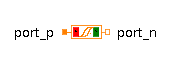Complex Constant Vector - MapleSim Help

Complex Constant Vector

Generate a complex constant vectorDescription The Complex Constant Vector block has a vector output of constant complex values. The value for a complex vector parameter is entered using a list of values. Each value may be entered using the complex constructor, for example, [Complex(3,4),Complex(5,6)], or using I for the imaginary unit, [3+4*I,5+6*I]. If a purely real value is entered, the real value will be converted to its complex equivalent.Equations $y=k$Connections

 Name Description Modelica ID $y$ Complex output vector yParameters

 Name Default Units Description Modelica ID $k$ Complex vector k